# Comprehensive Guide to LSTM & RNNsIn the current scenario of data and science, neural networks are emerging with the ability to rapidly perform tasks. This article will take a look at LSTM (long short-term memory) as one of the variants of neural networks. It will also shed light on the LSTM model and showcase how to implement the same in different situations.

## What is LSTM?

Long short-term memory (LSTM) deals with complex areas of deep learning. It has to do with algorithms that try to mimic the human brain to analyze the relationships in given sequential data. LSTM deep learning architecture can easily memorize the sequence of the data. It also eliminates unused information and helps with text classification.

LSTMs are one of the two special recurrent neural networks (RNNs) including usable RNNs and gated recurrent units (GRUs). RNN is a broader concept. Here’s an image depicting the basic functionality of these networks.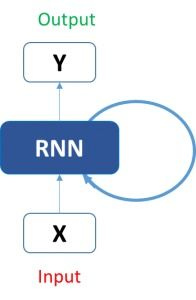Image source: Analyticsvidhya

LSTM is capable of analyzing data and learning long-term dependencies, especially in sequence prediction problems. It can process the entire data sequence apart from single data and images. This aids in machine translation, speech recognition, and more.

## Understanding the LSTM model

The main role of an LSTM model is controlled by a memory cell known as the ‘cell state’ that maintains its state over time. This is a horizontal line that runs through the top of the diagram below. It can be seen as a conveyor belt that carries the information flow.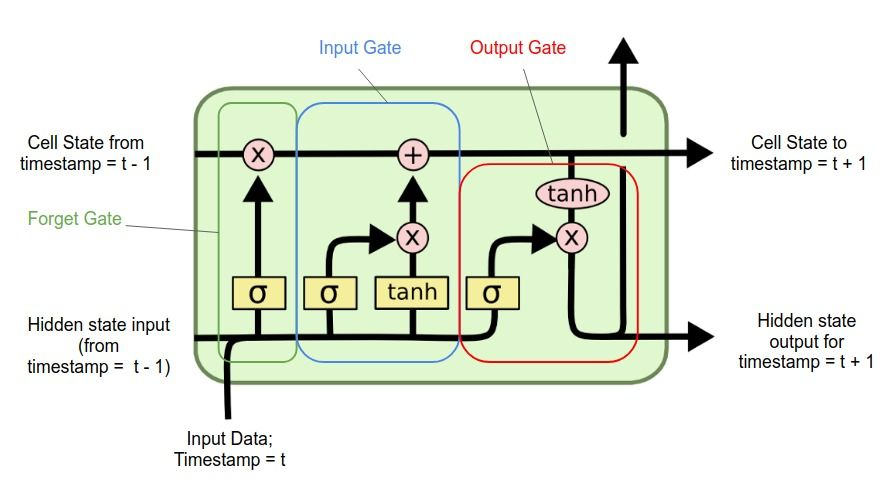Image source: Medium

The cell state and the hidden state are the two data states maintained in an LSTM model. An LSTM cell returns the hidden state for a single-time step which is the latest one.

A general LSTM neural network comprises the following components:

• Forget gate
• Input gate
• Output gate

The following diagram better depicts the LSTM network model.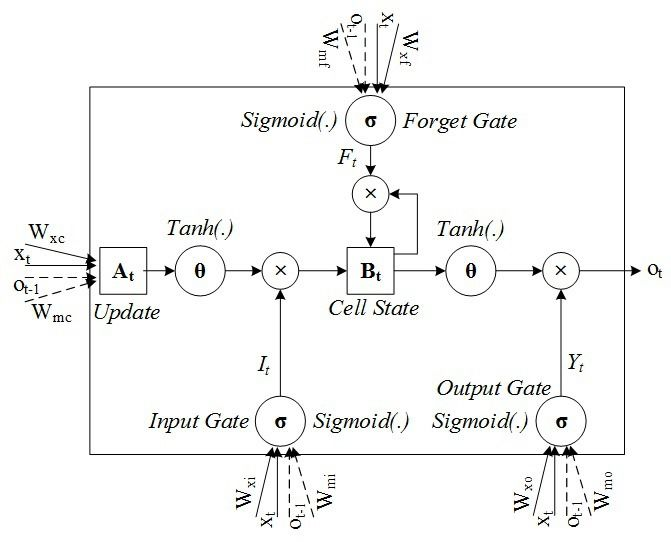Image source: Researchgate

### Forget gate

As discussed, LSTM recognizes and memorizes the information flowing inside the network. The forget gate is responsible for discarding the information that is not required to learn about the predictions.

Forget gate decides if the information can pass through the different layers of the network. It takes two types of input from the network:

• Information from the previous layers
• Information from the presentation layer.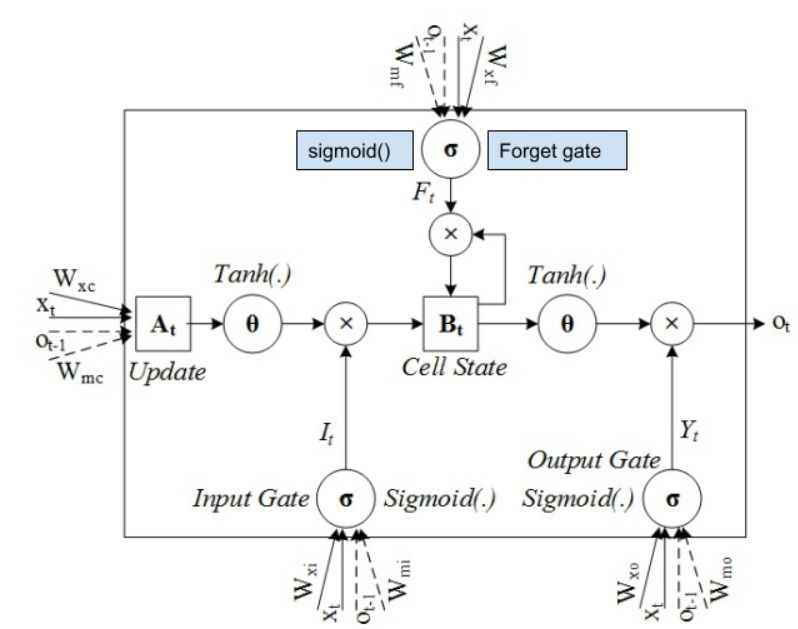Image source: Analyticsindiamag

The above diagram depicts the circuit of the forget gate, where ‘x’ and ‘h’ are the required information. The information passes through the sigmoid function and gets eliminated from the network if it goes towards ‘zero’.

### Input gate

Input gate decides the importance of the information by updating the cell state. It measures the integrity and importance of the information for developing predictions.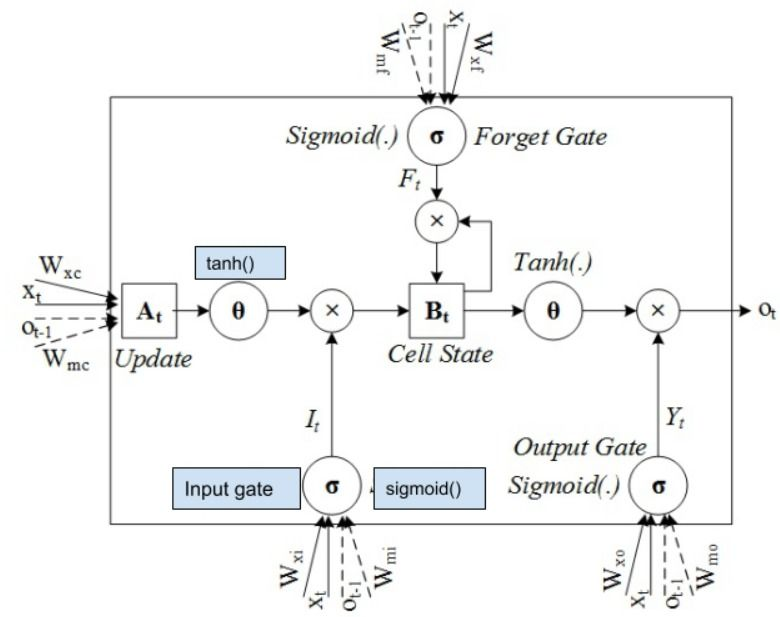Image source: Analyticsindiamag

The information passes through the sigmoid and the tanh functions. The tanh eliminates the bias of the network and the sigmoid determines the weight of the information.

### Cell state

The correct information passes through the cell state. Once here, the output of the input gate and forget gate is multiplied by each other.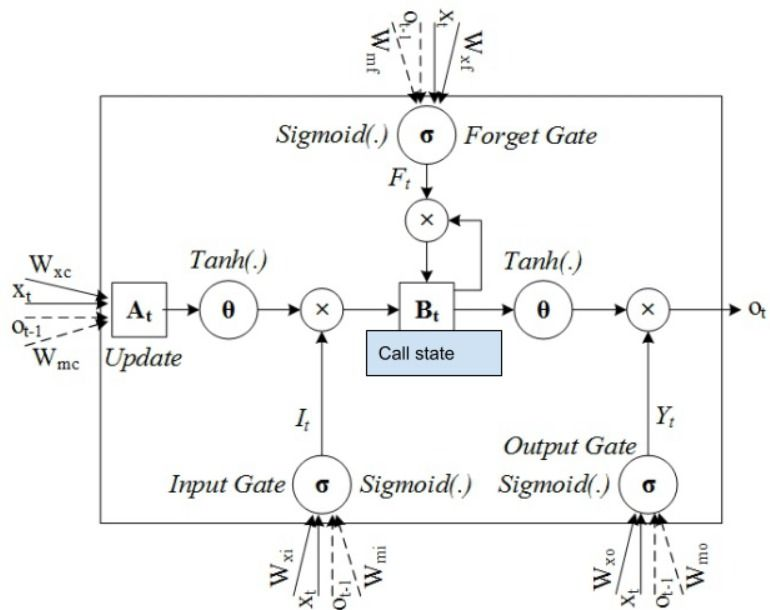Image source: Analyticsindiamag

### Output gate

The output gate is the last gate of the circuit. It decides the next hidden state of the network. The updated cell from the cell state goes to the tanh function and gets multiplied by the sigmoid function of the output state.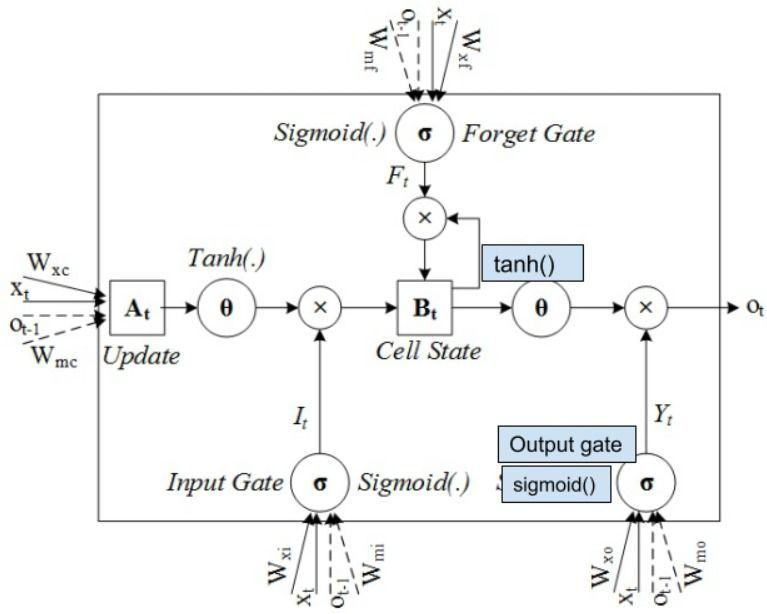Image source: Analyticsindiamag

## Why implement the LSTM neural network?

Text modeling focuses on preprocessing tasks and modeling tasks that create data sequentially. A few examples of text modeling processes are stop word elimination, POS tagging, and text sequencing. These display the results by feeding data into an LSTM model according to a predefined pattern.

Since LSTM eliminates unused information and memorizes the sequence of information, it’s a powerful tool for performing text classification and other text-based operations. It saves significant cost and time. It changes its type as hidden layers and different gates are added to it. In the BI LSTM (bi-directional LSTM) neural network, two networks pass information oppositely.

## Implementing the LSTM model using different approaches

### PyTorch LSTM

PyTorch is an open-source machine learning (ML) library developed by Facebook’s AI Research lab. Although quite new, it’s steadily becoming popular.

A specific attribute of PyTorch LSTM is that it expects all inputs to be 3D tensors. There are three axes according to the conditions:

• Sequence
• Indexing the instances in the mini-batch
• Indexing the elements of the input

Here’s an example.

#### Input: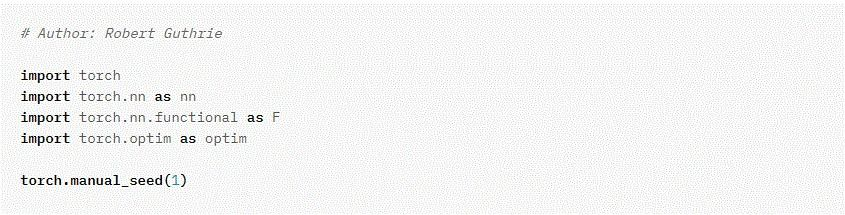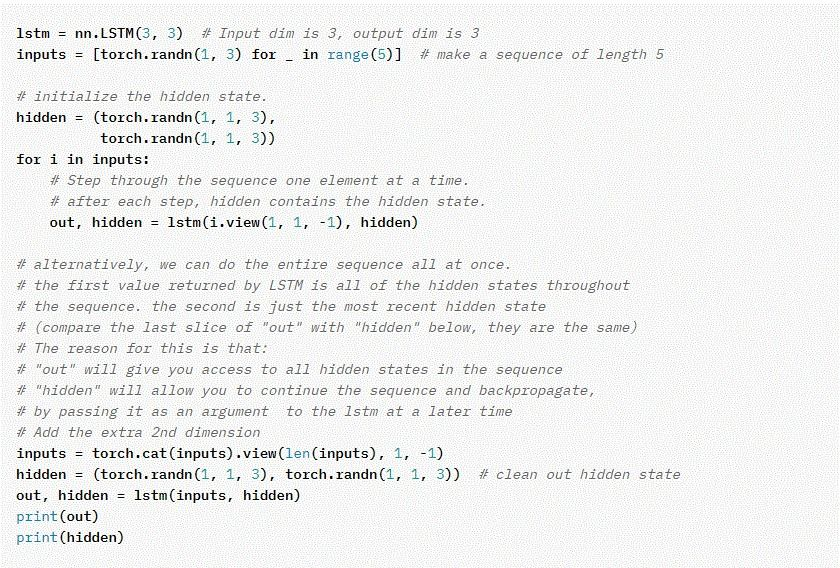#### Output: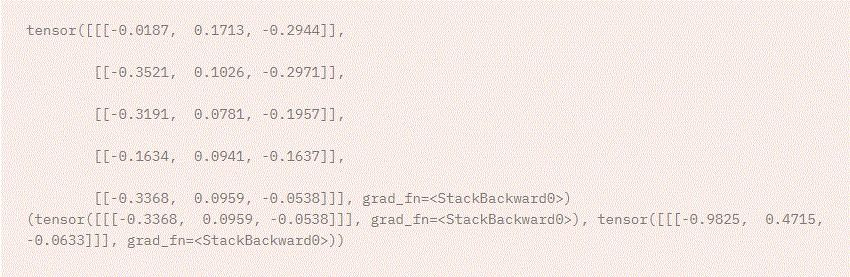Image source: Pytorch.org

### Keras LSTM

Here, we will use the LSTM network to classify MNIST data. Below are the steps to implement the LSTM model through Keras.

#### Importing the important modules: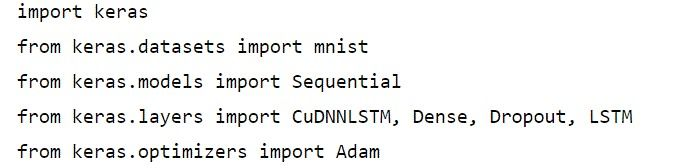#### Importing and preprocessing the MNIST data: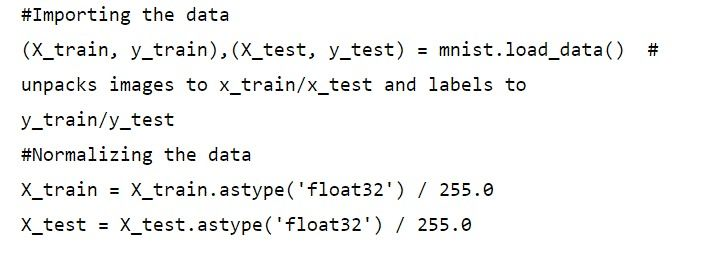#### Creating the LSTM network: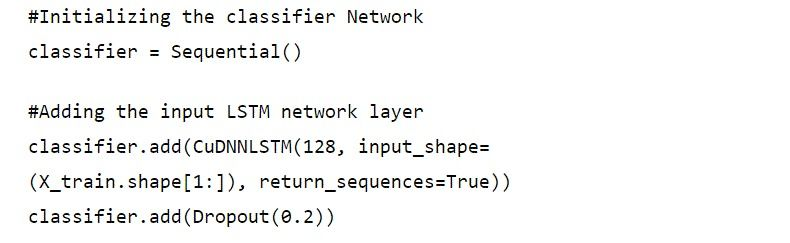Here’s an example to better understand the Keras LSTM.

#### Compiling the LSTM network and inserting the data: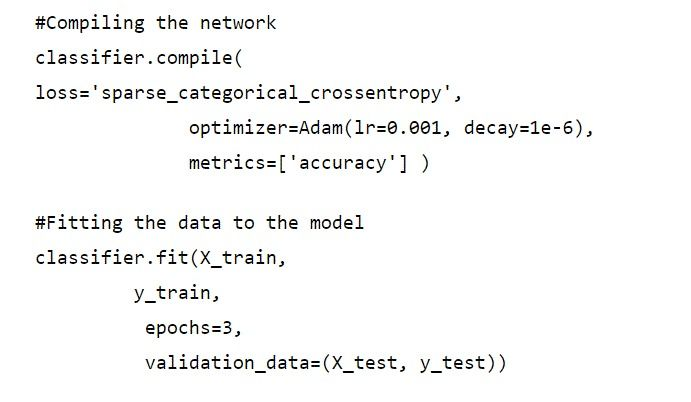#### Output: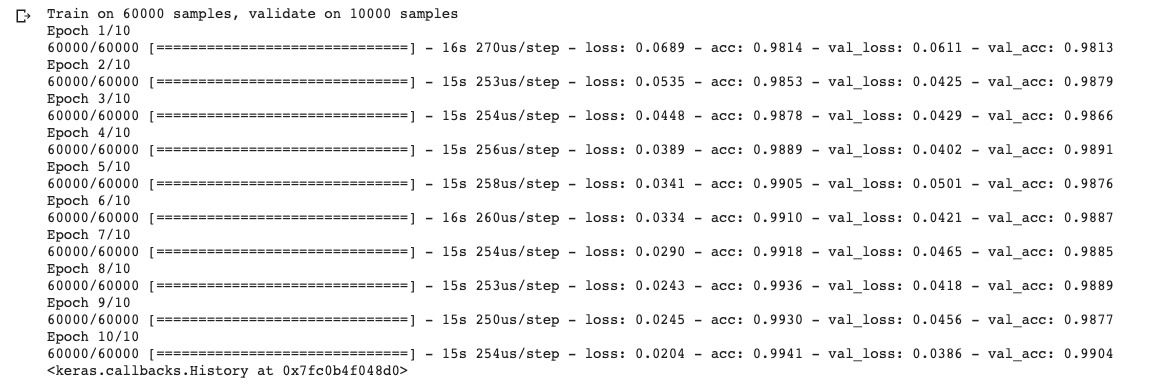#### Checking accuracy: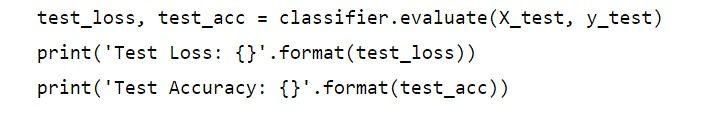#### Output: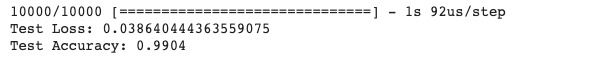Image source: Analyticsindiamag

### CNN LSTM

Convolutional neural network (CNN) is a feedforward neural network that is generally used in natural language processing (NLP) and image processing. It can be easily applied to time-series forecasting. Such architecture can improve the efficiency of model learning and, thus, reduce the number of parameters.

CNN is composed of two parts:

1. The pooling layer
2. The convolution layer

Each convolution layer contains multiple convolution kernels. The features of the data are extracted after the operation on the convolution layer. However, these features are very high. To address the issue, a pooling layer is added to the convolution later in order to decrease the feature dimension.

The CNN LSTM model is widely used in feature engineering. To understand this hybrid model better, let’s take an example of a stock forecasting model.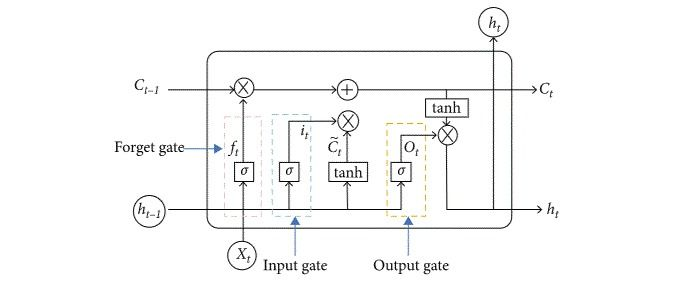Image source: Hindawi

The above diagram depicts the CNN LSTM model. It includes an input layer, a pooling layer, a convolution layer, a hidden LSTM layer, and a full connection layer.

Let’s define a CNN LSTM model in Keras by defining the CNN layers and then defining them in the output layers.

There are two ways to define the entire model:

#### Approach 1:

Define the CNN model first and add it to the LSTM model by wrapping the entire sequence of the CNN layers in a TimeDistributed layer.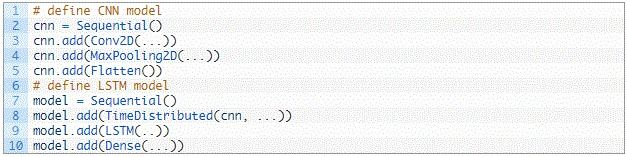#### Approach 2:

Wrap each layer in the CNN model in a TimeDistributed layer when adding the latter to the main model.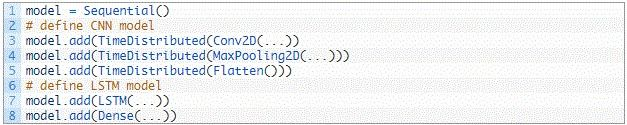Image source: Machinelearningamastery

## TensorFlow LSTM

Google’s TensorFlow is an end-to-end open-source platform for machine learning. It offers a comprehensive ecosystem of libraries, tools, and resources to let researchers develop and deploy ML-powered solutions.

Here’s an example that illustrates how to implement the LSTM model in TensorFlow.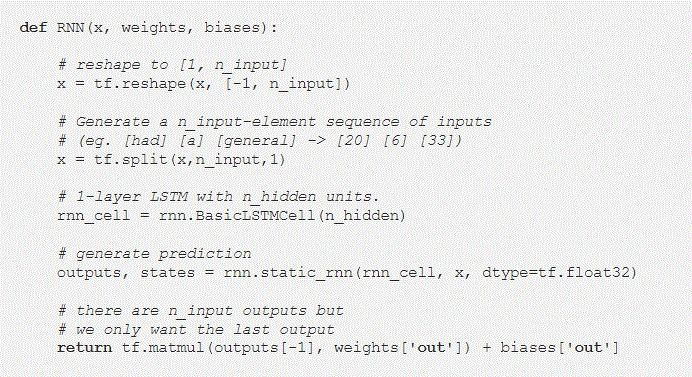Image source: Towardsdatascience

The LSTM model is a commonly used network that deals with sequential data like audio data, time-series data, and prediction. It’s possible to make multiple edits to the data or to the LSTM model itself, which can yield valuable insights and improve developers’ efficiency.### Press

What's up with Turing? Get the latest news about us here.### Blog